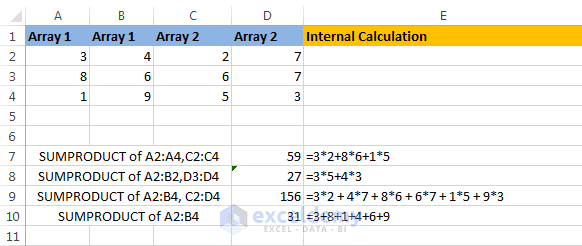# SUMPRODUCT() function in Excel

## SUMPRODUCT function: Syntax

SUMPRODUCT (array1, [array2], [array3], …)

## SUMPRODUCT function: Example

Example: =SUMPRODUCT(A2:B4, C2:D4). This example say that components (A2, A3, A4, B2, B3, and B4) of Array1 (A2:B4) will be multiplied with the components (C2, C3, C4, D2, D3, and D4) of Array2 (C2:D4) and then summed. So the calculation will be: A2*C2+ A3*C3+ A4*C4+ B2*D2+ B3*D3+ B4*D4.

The following figure shows some real-time examples.Some product real time examples.

## SUMPRODUCT function: Caution

• The array arguments must have the same dimensions. If they do not have the same dimensions, SUMPRODUCT function will return the #VALUE! error.
• SUMPRODUCT treats not numeric array values as zeros.